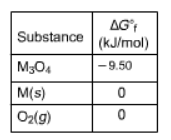# Problem: Consider the decomposition of a metal oxide to its elements, where M represents a generic metal.                                     M    3O4 (s) ⇌ 3 M (s) + 2 O2 (g) i) What is the standard change in Gibbs energy for the reaction, as written, in the forward direction?ii) What is the equilibrium constant of this reaction, as written, in the forward direction at 298 K?iii) What is the equilibrium pressure of O2 (g) over M (s) at 298 K?

###### FREE Expert Solution
84% (418 ratings)
###### FREE Expert Solution

i)

$ΔG^o_{rxn} = products - reactants$

$ΔG^o_{rxn} = [(3 mole M)(0 kJ/mol) + (2 mol O2)(0 kJ/mol)] - [(1 mole M_3O_4)(-9.50 kJ/mol)] = 9.50 kJ$

84% (418 ratings)###### Problem Details

Consider the decomposition of a metal oxide to its elements, where M represents a generic metal.

M    3O(s) ⇌ 3 M (s) + 2 O(g)

i) What is the standard change in Gibbs energy for the reaction, as written, in the forward direction?

ii) What is the equilibrium constant of this reaction, as written, in the forward direction at 298 K?

iii) What is the equilibrium pressure of O(g) over M (s) at 298 K?